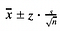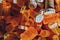# The Power of Statistics

Statistics is a branch of mathematics that is often overlooked. When the word “statistics” comes in mind, the average person may think of an MLB player’s batting average, or an NBA player’s field goal percentage. However, the reality is that statistics offers so much more than a simple percentage of shots that a player made throughout a season. It has the power that few other branches of mathematics contain: the power of generating definitive conclusions.Photo by Chris Liverani on Unsplash

# Confidence Intervals

Let’s say that a large, public university wants to estimate the average SAT score of all of its admitted students. It’s a relatively large school, so the administrators do not have the time to ask each student their score. They begin by randomly selecting 100 students, and then they calculate the sample mean. This sample mean is what will be used to approximate the mean SAT score for the entire university. Obviously, the sample mean is only one value, so the chance that its value is equivalent to the population mean is extremely small. In addition, if we randomly select another 100 students and calculate that sample mean, it is high likely the two sample means will be different. So, the confidence interval solves this issue by taking into account the sample data and creating a set of values which is likely to contain the actual population mean. There are different formulas for these confidence intervals depending on the sample size, if there are one or two samples being compared, or if the samples contain values that are proportions or means. But for this particular example, the upper bound of the confidence interval is found by adding the confidence level value, z, times the sample standard deviation divided by the square root of the sample size to the sample mean. The lower bound is found by subtracting the previous value from the sample mean. The resulting confidence interval equation is then:One-Sample Mean Confidence Interval Equation

# Hypothesis Testing

Another useful statistical method is the hypothesis test. These tests are useful in situations where perhaps a company is trying to determine the efficiency of their product. In any hypothesis test, there is a null hypothesis and an alternative hypothesis. The null hypothesis is always the status quo: what is presumed to be true. The alternative hypothesis is then the hypothesis that we want to establish. For example, let’s imagine a scenario where a high school student wants to test if a coin is biased or not (if the chance of being heads is not exactly 50%). The null hypothesis would be that the proportion of heads equals 50%, and the alternative hypothesis would be the proportion of heads is not equal to 50%. Like confidence intervals, there are also different formulas for these hypothesis tests depending on different situations. However, the result of these formulas, or the test statistics, always come out to a decimal value, with which we can calculate a p-value. The formal definition of the p-value is the probability of attaining results as extreme or more extreme than the observed test statistic, provided that the null hypothesis is true (this is important). A lower p-value would then result in a higher chance of rejecting the null hypothesis, and a higher p-value would result in a lower chance of rejecting the null hypothesis. In most standard hypothesis tests, the tipping point is if the p-value is less than 0.05. If the p-value is less than 0.5, that means we reject the null hypothesis, and if the p-value is greater than 0.5, we we fail to reject it.Photo by Haley Lawrence on Unsplash

## Thanks For Reading!

I hope you enjoyed my first article! If you are interested in my work, please consider following.

17 || High School Junior || stats & math